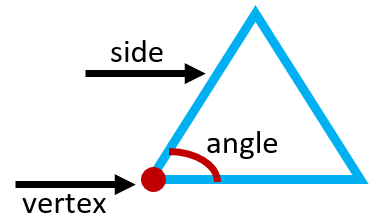# Math Worksheets Land

Math Worksheets For All Ages

# Math Worksheets Land

Math Worksheets For All Ages

# The Attributes of Two-dimensional Figures Worksheets

In this section we are focused on understand the vocabulary and properties that help us describe a figure and understand how to compare two different figures. This section builds upon a topic that you have seen for a few years now in the curriculum. I also find it very helpful to work with students on the concept of contrast and comparisons of two to three things. This does not need to be object based, it can just be those fun pictures that you will find in the comic section of any paper. It is all about helping students be able to spot likenesses and differences that are not overtly obvious. These worksheets and lessons will help students be able to name 2D shapes based on the number of sides and vertices.

### Aligned Standard: Grade 5 Geometry - 5.G.3

• Answer Keys - These are for all the unlocked materials above.

### Homework Sheets

As one student wrote in, "These worksheets are like looking for a needle in the haystack."

• Homework 1 - Choose the correct word that describes the shape. If the lines of the shape were to continue, they would never run into each other.
• Homework 2 - It looks like a letter a 'Trapezoid' ,but we can’t be sure unless all the lines are parallel. Since the lines will never run into each other, we know all lines are parallel.
• Homework 3 - Define and draw the shape of Rhombus.

### Practice Worksheets

It is really neat to see what some students come up with since there are multiple answers for a few of these.

• Practice 1 - Draw shapes that match all these criteria and name the shape.
• Practice 2 - Rhombus: A 4-sided flat shape with straight sides where all sides has equal length. Also opposite sides are parallel and opposite angles are equal.
• Practice 3 - Choose the correct word that describes the shape. If the lines of the shape were to continue, they would never run into each other.

### Math Skill Quizzes

The quizzes really cover everything that I have ever seen on this standard in one question. Is it a bit much?

• Quiz 1 - Identify the shape and record the correct number of corners and sides for each plane figure on the lines below.
• Quiz 2 - Name and circle the different shape.
• Quiz 3 - Color the shapes and write their names.

### What Are the Common Attributes of Two-dimensional Figures?

Students in later elementary school often face the concept of learning how to go about describing two-dimensional figures. The first thing we need to become familiar with is the vocabulary that helps us make sense of all these attributes. Any figure where all the sides meet is called a closed figure. The line segments that make up 2D shapes are called sides. The point that the sides or corners of a figure touch are called vertex. The measure of the space between two sides that meet at a vertex is called an angle. Take a look at this vocabulary in action in this diagram:Two-dimensional figures are described through their attributes, and there are attributes in these figures that are common. A 2D figure lies on the two axes, the X-axis, and the Y-axis. Hence the name two-dimensional figure. Which also means that there are only two measures to consider at this point which are the longest side (length) and the opposite side (height). Since we are looking at only 2D figures, thickness (width) is not a consideration that we need to make. Because of this 2D figures have an area but lack volume. The number of sides, angles (corners), and vertices dictate how these shapes are classified. There are some shapes that share the same qualities but differ by another attribute. For example, there are different sub-categories of shapes such as rhombus and rectangle with common attributes, i.e., 4 sides. These attributes can also be extended to quadrilaterals. The next topic will go all into how to classify and eventually name these shapes, right now we just want to be able to identify what is the same and what is different between these structures.

One of the most common and basic attributes of a 2D figure is that it has a line segment, with the exception of a circle. A line segment has endpoints, i.e., a starting point and an ending point. The order of the endpoints doesn’t matter in the 2D figures. Take the example of an oval and a circle. Both have curves and are entire round shape figures. The only difference between them is that a circle has one radius throughout.

Unlock all the answers, worksheets, homework, tests and more!
Save Tons of Time! Make My Life Easier Now

## Thanks and Don't Forget To Tell Your Friends!

I would appreciate everyone letting me know if you find any errors. I'm getting a little older these days and my eyes are going. Please contact me, to let me know. I'll fix it ASAP.